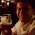Friday, May 15, 2009

Thermoelectric Temperature Controller - Part 4

As promised, here is the program code for use with the PICAXE and it's programming editor: Program Code

The verbal description of this program is this:
1. Configure the display
2. Read the temperature from the LM34
3. Display the temperature on the LED display
4. If the temperature set point is below the current temp, then perform a PWM command and turn on the Peltier device. The amount of drive is proportional to the error between the set point and current temp.
5. If the temperature set point is above the current temp, then turn the PWM off.
6. If at any point, the temperature set point button is pressed, flash the set point LED and enter the set temp mode. Read the temperature set point from the potentiometer. Press and release the button quickly to exit the set temp mode.
7. Keep looping.Some points within the program that may not be so obvious. First, when we read the temperature from the LM34, the picaxe reads the analog voltage and converts it to a digital value with ten bits of resolution. This means that the value has 2^10 or 1024 divisions. The digital value can then be calculated back into a voltage in mV within our program. The ADC is converting based on a 5V range, or 5000 mV. This means that a digital value of 0 is equal to 0 mV and a digital value of 1024 is equal to 5000 mV. Taking this further, each digital division is equivalent to 5000/1024, or 4.88 mV. By the way, 4.88 mV is the same as 0.488 deg F, so the resolution of our program is ~0.5 deg F. You can see from the program that when we mulitply the digital value by 4.88, we will in essence be calculating the temperature in deg F mulitplied by ten. Since the temperature is read in tens of millivolts, the actual whole number value of the temperature can be read once we divide TE_Temp_10 by 10.

I will update this discussion of my code at a later time, hopefully the code will speak for itself. Enjoy!

1.You're making great progress. I never get as far as fooling around with the control electronics. My work is primarily what's inside the TEC, to a lesser extent with the thermal assembly, and very little about the control electronics.

The efficiency of the heat transfer path is very important. That means using the right thermal interface materials, appropriate heat sinks and fans, etc.

One trick I often see neglected is to over-spec the thermoelectric parts of the design, then run them well below their maximum power capacity. A TEC will pump an amount of heat that varies linearly with the amount of electrical current, but its power consumption goes with the square of current. One module at max current pumps the same amount of heat as four modules at one-quarter current, but the latter case uses only half the power to do so. Or something like that. It's been a while since I looked at the math.

2.Jeff -
Thanks for the info - I did neglect this trick in the project. I will check into the math, I appreciate the help!

3.Hi!
Very interesting project. I would like to build a TEC controller that can be accessed via RS232 or ethernet to be able to set and read out the temperature. Do you think your controller can be extended with such capabilities? I'm beginner with microcontrollers but not so much beginner with electronics... Thanks in advance. Janos